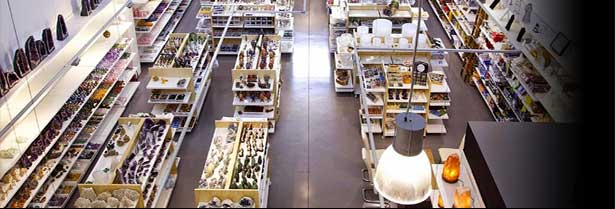### Specialized store in wholesale of mineralsQuantity:

Quantity:

Quantity:

Quantity:

Quantity:

Quantity:

Quantity:

Quantity:

Quantity:

Quantity:

Quantity:

Quantity:

Quantity:

Quantity:

Quantity:

Quantity:

Quantity:

Quantity:

Quantity:

Quantity:

Quantity:

Quantity:

Quantity:

Quantity:

Quantity:

Quantity:

Quantity:

Quantity:

Quantity:

Quantity:

Quantity:

Quantity:

Quantity:

Quantity:

Quantity:

Quantity:

Quantity:

Quantity:

Quantity:

Quantity:

Quantity:

Quantity:

Quantity:

Quantity:

Quantity:

Quantity:

Quantity:

Quantity:

Quantity:

Quantity:

Quantity:

Quantity:

Quantity:

Quantity:

Quantity:

Quantity:

Quantity:

Quantity:

Quantity:

Quantity:

Quantity:

Quantity:

Quantity:

Quantity:

Quantity:

Quantity:

Quantity:

Quantity:

Quantity:

Quantity:

Quantity:

Quantity:

Quantity:

Quantity:

Quantity:

Quantity:

Quantity:

Quantity:

Quantity:

Quantity:

Quantity:

Quantity:

Quantity:

Quantity:

Quantity:

Quantity:

Quantity:

Quantity:

Quantity:

## Turkish Eye Bracellet. Set 5un

 Colour: MulticolourRedDark Green

Quantity:

## Turkish Eye Bracellet. Set 5un

 Colour: MulticolourRedDark Green

Quantity:

## Turkish Eye Bracellet. Set 5un

 Colour: MulticolourRedDark Green

Quantity:

Quantity:

Quantity:

## White Flower Pendant. Set 5un

Quantity:

Quantity:

Quantity:

Quantity:

Quantity:

Quantity:

 Displaying 1 to 100 (of 3991 products) Result Pages:  1  2  3  4  5  6  7  8  9  10 ...  [Next >>]